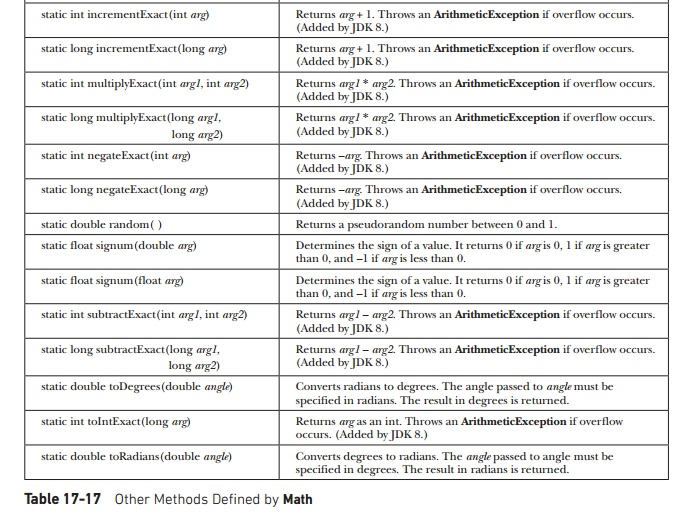Home | | Web Programming | Math - java.lang

# Math - java.lang

The Math class contains all the floating-point functions that are used for geometry and trigonometry, as well as several general-purpose methods.

Math

The Math class contains all the floating-point functions that are used for geometry and trigonometry, as well as several general-purpose methods. Math defines two double constants: E (approximately 2.72) and PI (approximately 3.14).

Trigonometric Functions

The following methods accept a double parameter for an angle in radians and return the result of their respective trigonometric function:

Method : Description

static double sin(double arg) : Returns the sine of the angle specified by arg in radians.

static double cos(double arg) : Returns the cosine of the angle specified by arg in radians.

static double tan(double arg) : Returns the tangent of the angle specified by arg in radians.

The next methods take as a parameter the result of a trigonometric function and return, in radians, the angle that would produce that result. They are the inverse of their non-arc companions.

Method : Description

static double asin(double arg) : Returns the angle whose sine is specified by arg.

static double acos(double arg) : Returns the angle whose cosine is specified by arg.

static double atan(double arg) : Returns the angle whose tangent is specified by arg.

static double atan2(double x, double y) : Returns the angle whose tangent is x/y.

The next methods compute the hyperbolic sine, cosine, and tangent of an angle:

Method : Description

static double sinh(double arg) : Returns the hyperbolic sine of the angle specified by arg.

static double cosh(double arg) : Returns the hyperbolic cosine of the angle specified by arg.

static double tanh(double arg) : Returns the hyperbolic tangent of the angle specified by arg.

Exponential Functions

Math defines the following exponential methods:Rounding Functions

The Math class defines several methods that provide various types of rounding operations. They are shown in Table 17-16. Notice the two ulp( ) methods at the end of the table. In this context, ulp stands for units in the last place. It indicates the distance between a value and the next higher value. It can be used to help assess the accuracy of a result.Miscellaneous Math Methods

In addition to the methods just shown, Math defines several other methods, which are shown in Table 17-17. Notice that several of the methods use the suffix Exact. These were added by JDK 8. They throw an ArithmeticException if overflow occurs. Thus, these methods give you an easy way to watch various operations for overflow.The following program demonstrates toRadians( ) and toDegrees( ):

class Angles {

public static void main(String args[]) { double theta = 120.0;

theta = 1.312;

System.out.println(theta + " radians is " + Math.toDegrees(theta) + " degrees.");

}

}

The output is shown here:

StrictMath

The StrictMath class defines a complete set of mathematical methods that parallel those in Math. The difference is that the StrictMath version is guaranteed to generate precisely identical results across all Java implementations, whereas the methods in Math are given more latitude in order to improve performance.

Compiler

The Compiler class supports the creation of Java environments in which Java bytecode is compiled into executable code rather than interpreted. It is not for normal programming use.

Study Material, Lecturing Notes, Assignment, Reference, Wiki description explanation, brief detail
Java The Complete Reference : The Java Library : Exploring java.lang : Math - java.lang |